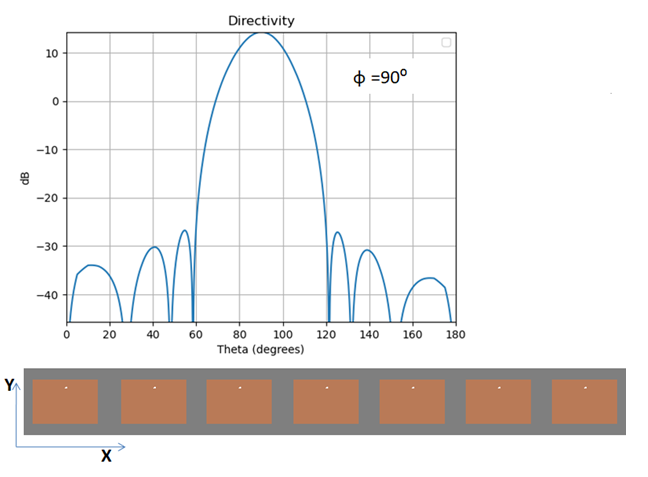# Antenna Design for 5G mmWave Arrays

By Hany A. Raouf, PhD         June 2020

The antenna design for 5G  mmWave arrays has the following important aspects: the beamforming and synthesizing the array, designing the antenna elements; designing the feeding network, the effect of the coupling between the adjacent antenna elements, and the effect of surrounded materials on the radiation characteristics of the antennas.

The beamform is designed in order to maximize each user’s received signal power while minimizing the interference signal power from the other users.

In this blog we will discuss the design of mmWave array of patches at 30 GHz . Figure 1(a) shows the single patch element with coaxial feeding line. The substrate material is RT-duroid 5880 with 2.33 dielectric constant. The location of the feeding point can be adjusted to produce circular polarization which can be left hand circular (LHC) or right hand circular (RHC) based on the position of the feeding point. In addition changing the feeding point position improves the return loss to values between -10 dB and -15 dB, as we discussed in .

The S11 is simulated using FDTD for the single patch antenna of Figure1 and the result is shown in Figure 1(b).  The result shows resonant frequency at 30 GHz. The radiation pattern of the of the single patch in the YZ plane is shown in Figure 2.

Now we use the above patch antenna in synthesizing of several arrays with non-uniform amplitude distribution. We can use different methods based on the beamforming requirements. In the following examples it is required to design side lobe levels (SLL) below -30 dB and half power beamwidth about 10 degrees.  We used uniform distance λ/2 between the patches.

Our code takes the shape of the radiation pattern or the beamform requirements as input and calculate the array parameters, e.g. spaces between the elements, amplitudes  as outputs. Then we simulate the whole array to consider the coupling effect between the elements.Figure 3: Directivity of a linear non-uniform amplitude array of 7 elements at f=30 GHz. The results show HPBW of 20 degrees.

Figure 4: Directivity of a linear non-uniform amplitude array of 14 elements at f=30 GHz. The results show HPBW of 10 degrees.

Figure 4 shows that the 14 elements array satisfies the required beamforming specifications.

Figure 5: Directivity of a linear non-uniform amplitude array of 21 elements at f=30 GHz. The results show HPBW of 6.8 degrees.

Figure 6: Comparison of the total radiation pattern and the array factor of a linear non-uniform amplitude array of 21 elements of Figure 4.

Figure 7: Directivity of non-uniform amplitude array of 21 elements of Figure 5.

Figure 8 shows the directivity polar plot for scanning angle θ= 30 degrees as an example.

Figure 8: Directivity of a linear non-uniform amplitude array of 21 elements at f=30 GHz when scan to angle 30 degrees.

HASystem Tech can choose which method/software is the best for your design.

Simulation of massive array of tightly coupled antennas with the feeding network over an UWB, e.g. 20 – 50 GHz is challenging. HAsystem Tech solve such kind of problems using techniques that we had developed such as domain decomposition.